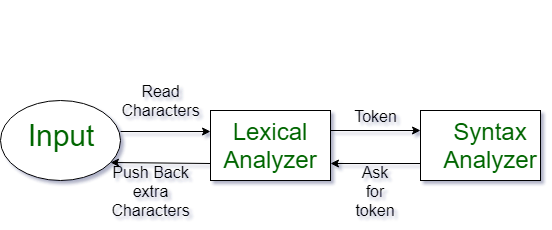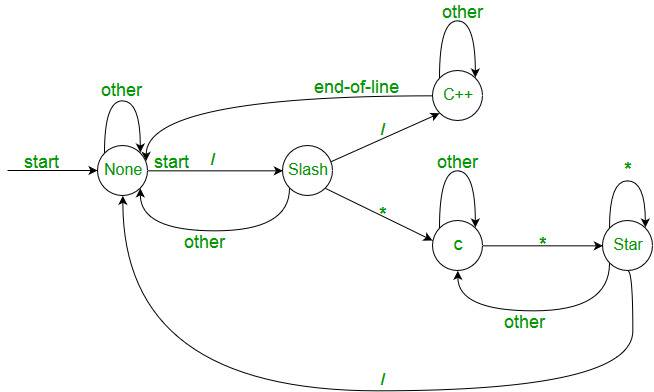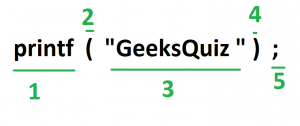Open in App
Not now

# Introduction of Lexical Analysis

• Difficulty Level : Easy
• Last Updated : 04 Jan, 2023

Lexical Analysis is the first phase of the compiler also known as a scanner. It converts the High level input program into a sequence of Tokens.

• Lexical Analysis can be implemented with the Deterministic finite Automata.
• The output is a sequence of tokens that is sent to the parser for syntax analysisWhat is a token? A lexical token is a sequence of characters that can be treated as a unit in the grammar of the programming languages. Example of tokens:

• Type token (id, number, real, . . . )
• Punctuation tokens (IF, void, return, . . . )
• Alphabetic tokens (keywords)
```Keywords; Examples-for, while, if etc.
Identifier; Examples-Variable name, function name, etc.
Operators; Examples '+', '++', '-' etc.
Separators; Examples ',' ';' etc```

Example of Non-Tokens:

• Comments, preprocessor directive, macros, blanks, tabs, newline, etc.

Lexeme: The sequence of characters matched by a pattern to form the corresponding token or a sequence of input characters that comprises a single token is called a lexeme. eg- “float”, “abs_zero_Kelvin”, “=”, “-”, “273”, “;” .

How Lexical Analyzer works-

1. Input preprocessing: This stage involves cleaning up the input text and preparing it for lexical analysis. This may include removing comments, whitespace, and other non-essential characters from the input text.
2. Tokenization: This is the process of breaking the input text into a sequence of tokens. This is usually done by matching the characters in the input text against a set of patterns or regular expressions that define the different types of tokens.
3. Token classification: In this stage, the lexer determines the type of each token. For example, in a programming language, the lexer might classify keywords, identifiers, operators, and punctuation symbols as separate token types.
4. Token validation: In this stage, the lexer checks that each token is valid according to the rules of the programming language. For example, it might check that a variable name is a valid identifier, or that an operator has the correct syntax.
5. Output generation: In this final stage, the lexer generates the output of the lexical analysis process, which is typically a list of tokens. This list of tokens can then be passed to the next stage of compilation or interpretation.• The lexical analyzer identifies the error with the help of the automation machine and the grammar of the given language on which it is based like C, C++, and gives row number and column number of the error.

Suppose we pass a statement through lexical analyzer – a = b + c ;                It will generate token sequence like this: id=id+id;                 Where each id refers to it’s variable in the symbol table referencing all details For example, consider the program

```int main()
{
// 2 variables
int a, b;
a = 10;
return 0;
}```

All the valid tokens are:

```'int'  'main'  '('  ')'  '{'  'int'  'a' ','  'b'  ';'
'a'  '='  '10'  ';' 'return'  '0'  ';'  '}'```

Above are the valid tokens. You can observe that we have omitted comments. As another example, consider below printf statement.There are 5 valid token in this printf statement. Exercise 1: Count number of tokens :

```int main()
{
int a = 10, b = 20;
printf("sum is :%d",a+b);
return 0;
}
Answer: Total number of token: 27.```

Exercise 2: Count number of tokens : int max(int i);

• Lexical analyzer first read int and finds it to be valid and accepts as token
• max is read by it and found to be a valid function name after reading (
• int  is also a token , then again i as another token and finally ;
``` Answer:  Total number of tokens 7:
int, max, ( ,int, i, ), ;```

Below are previous year GATE question on Lexical analysis. https://www.geeksforgeeks.org/lexical-analysis-gq/ Please write comments if you find anything incorrect, or you want to share more information about the topic discussed above

My Personal Notes arrow_drop_up
Related Articles